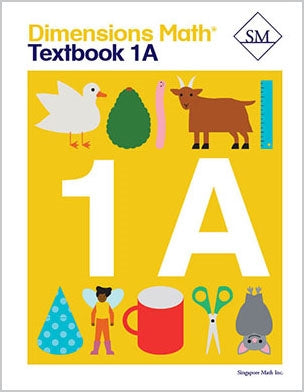# Dimensions Math Textbook 1A

\$ 12.00

Dimensions Math® Textbook 1A

Chapter 1: Numbers to 10

Lesson 1: Numbers to 10
Lesson 2: The Number 0
Lesson 3: Order Numbers
Lesson 4: Compare Numbers
Lesson 5: Practice

Chapter 2: Number Bonds

Lesson 1: Make 6
Lesson 2: Make 7
Lesson 3: Make 8
Lesson 4: Make 9
Lesson 5: Make 10 — Part 1
Lesson 6: Make 10 — Part 2
Lesson 7: Practice

Lesson 1: Addition as Putting Together
Lesson 4: Addition with Number Bonds
Lesson 5: Addition by Counting On
Lesson 8: Practice

Chapter 4: Subtraction

Lesson 1: Subtraction as Taking Away
Lesson 2: Subtraction as Taking Apart
Lesson 3: Subtraction by Counting Back
Lesson 4: Subtraction with 0
Lesson 5: Make Subtraction Stories
Lesson 6: Subtraction with Number Bonds
Lesson 8: Make Addition and Subtraction Story Problems
Lesson 9: Subtraction Facts
Lesson 10: Practice
Review 1

Chapter 5: Numbers to 20

Lesson 1: Numbers to 20
Lesson 2: Add or Subtract Tens or Ones
Lesson 3: Order Numbers to 20
Lesson 4: Compare Numbers to 20
Lesson 6: Subtraction
Lesson 7: Practice

Lesson 1: Add by Making 10 — Part 1
Lesson 2: Add by Making 10 — Part 2
Lesson 3: Add by Making 10 — Part 3
Lesson 4: Addition Facts to 20
Lesson 5: Practice

Chapter 7: Subtraction Within 20

Lesson 1: Subtract from 10 — Part 1
Lesson 2: Subtract from 10 — Part 2
Lesson 3: Subtract the Ones First
Lesson 4: Word Problems
Lesson 5: Subtraction Facts Within 20
Lesson 6: Practice

Chapter 8: Shapes

Lesson 1: Solid and Flat Shapes
Lesson 2: Grouping Shapes
Lesson 3: Making Shapes
Lesson 4: Practice

Chapter 9: Ordinal Numbers

Lesson 1: Naming Positions
Lesson 2: Word Problems
Lesson 3: Practice
Review 2

Dimensions Math® PreK-5 series features the progression, rigor, and pacing that define Singapore math. Throughout the series, five characters offer students suggestions on how to think about problems. They remind students of strategies they’ve learned and point out important information that encourages them to come up with their own solutions.

Textbook lessons begin with a task that allows students to apply their previous knowledge and learn through discussion. Once students have mastered a concept with the use of concrete and pictorial aids, they are ready to take on more abstract mathematical problem sets. They reach fluency by collecting various strategies along the way and applying them to new problems. Word problems give students a sense of math in real-world contexts.

Workbooks offer independent practice that follows a careful progression of exercise variation. Each textbook lesson includes a corresponding workbook exercise that starts with pictorial representation and progresses to more challenging abstract problems. Workbooks for PreK-2 are perforated.

Teacher’s Guides include lesson plans, mathematical background, games, helpful suggestions, and comprehensive resources for daily lessons. Lessons are laid out clearly and activities are designed for the whole class, small groups, and extension.

Textbooks and Workbooks do not include answer keys. Answers are in Teacher's Guides.
Textbook:
ISBN 9781947226043
pp 176